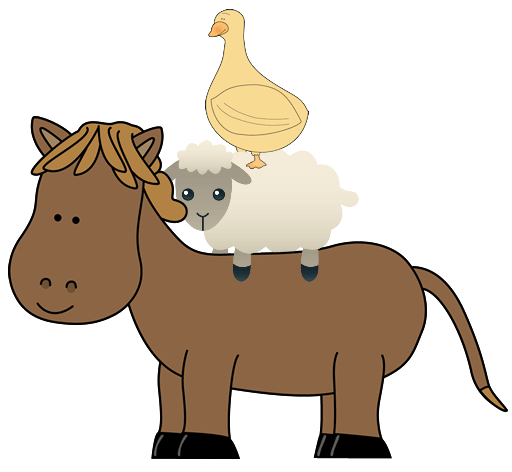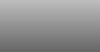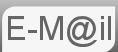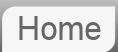# Answer to Riddle #24: The Market

24. You're a farmer. You're going to a market to buy some animals. On the market there are 3 types of animals for sale. You can buy: Horses for £10 each, goats for £1 each and ducks, you get 8 of these per bunch and each bunch costs £1. The aim is to acquire 100 animals at the cost of £100, what is the combination of horses, goats and duck that allows you to do this? (you must buy at least one of each.)

This puzzle was e-mailed to me by Shaun Ball, for the record this one took me about 20 minutes to solve by brute force, how long did it take you...?Before reading the answer can I interest you in a clue?

This is problem can be solved relatively easily by using a Brute Force technique, that is to say trying lots of combinations. There are some things you can do to speed up your working....
• We can have a maximum of 10 horses or we would have no money left.
• We can have a maximum of 12 bunches of ducks or we would have too many animals.
• A spread sheet might help
However there is a more eloquent approach (thanks to Gary Short):

This can be solved as a simultaneous equation, with three variables and two equations...

Our equations are:
1. 10*H + G + D/8 = 100 (pounds)
2. H + G + D = 100 (animals)
Subtracting equation #2 from equation #1 gives:
9H - 7D/8 = 0
OR....
9H = 7D/8
(at this point we can actually stop solving the equation as we know that ducks come in bunches of 8 so the feature D/8 in the equation actually represents the number of bunches of duck, therefore the equation is simply 9H = 7DB which easily gives us a solution. But we will continue....)

72H = 7D
Directly this equation is unsolvable as there are an infinite number of solutions. However, we have the restraint that both variables must be integers and that there H<100 & D<100.

Hence the only solutions is
H = 7 : D = 72 : G = 21

The answer is 7 horses, 21 goats and 9 bunches of ducks.

© Nigel Coldwell 2004 -  – The questions on this site may be reproduced without further permission, I do not claim copyright over them. The answers are mine and may not be reproduced without my expressed prior consent. Please inquire using the link at the top of the page. Secure version of this page.

PayPal
I always think it's arrogant to add a donate button, but it has been requested. If I help you get a job though, you could buy me a pint! - nigel